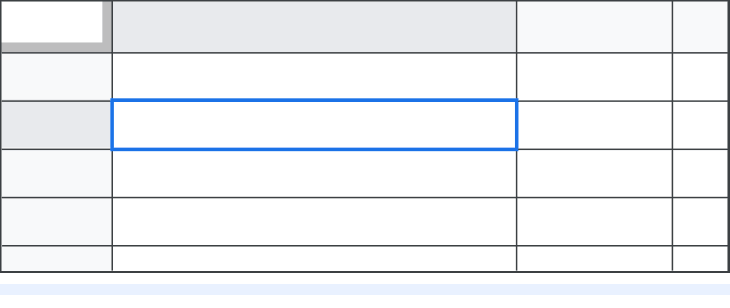You can use functions and formulas to automate calculations in Google Sheets.

Tip: If you’re already familiar with functions and formulas and just need to know which ones are available, go to Google Sheets function reference.

## Learn what a function is

A formula is anything that’s entered into a cell that begins with the equals character (=). For example:

=2+3

Formulas often include functions, but they're not required. The following example shows a formula that uses the IF and SUM functions to determine whether expenses are over or under budget:

=IF(SUM(A2:A)>C2,"Over Budget","Under Budget")

In the example below, expenses are over budget because \$350 is more than \$300.In the example above:

• A2:A includes all values in column A from row 2 to the bottom of the column.
• The formula assumes expenses are in column A and the budget is in cell C2.
• As you enter a value, a popup displays the projected output.
• The output changes when you change any value in cell C2 or column A.

## Get started with functions

• Functions usually perform calculations on values.
• Values can also be called arguments or inputs.
• The function can have zero, one, or multiple inputs.
• The inputs themselves may be values you type in, cells, or ranges of cells
• Some functions have optional inputs that have default values when no values are provided.

## Zero inputs

The function NOW takes zero inputs. It doesn’t need any information to tell the current day and time.

To try the function, type =NOW() into any spreadsheet cell, then press Enter.## One input

The function ABS takes one input, which must be either a single value or a single cell. For example:

=ABS(-5) or =ABS(B2)## Multiple inputs

The functions SUM and CONCAT take multiple inputs, which can be any combination of values or cell references. For example, =SUM(B1, 17, D1:D3) calculates the sum of the value in cell B1, the number 17, and the values in cells D1, D2, and D3.## Optional inputs

• The function ROUND takes either one or two inputs and rounds the first input.
• You can specify the decimal place to round in the second input.
• If the second input isn’t specified, the function rounds to the nearest whole number. This optional input is indicated with square brackets:

ROUND(value, [places])

For example:

=ROUND(123.456, 2):  This function rounds the number to 2 decimal places.

=ROUND(123.456):      This function rounds to the nearest whole number.## Learn what input a function needs

To find what input a function needs:

• Use the Google Sheets functions list. The list includes the names and descriptions of every input. For example:

VLOOKUP(search_key, range index, [is_sorted])

• Use function suggestions. Type the function name to get the list of functions, then select the function you want to learn about.
• You can click the arrow to check the input names or view all info about the function.
• Each input is highlighted in green as you enter values into the function.An output is the result of a function. For many functions, such as NOW, ABS, SUM, and ROUND, the result is a single value.

However, the output of some functions appears in multiple cells. For example, the result of the UNIQUE function is a list of the multiple, unique values from the input.

In this example, the input is a range of 7 cells and the output is 4 cells: